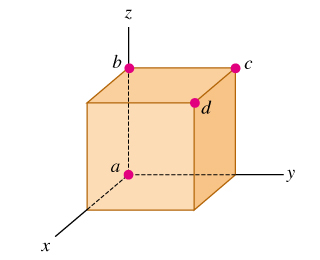# Problem: A cube is placed so that one corner is at the origin and three edges are along the x-, y-, and z -axes of a coordinate system (the figure ). Use vectors to answer the questions.Find the angle between the edge along the z-axis (line ab) and the diagonal from the origin to the opposite corner (line ad).Find the angle between line ac (the diagonal of a face) and line ad .

⚠️Our tutors found the solution shown to be helpful for the problem you're searching for. We don't have the exact solution yet.

###### Problem Details

A cube is placed so that one corner is at the origin and three edges are along the x-, y-, and z -axes of a coordinate system (the figure). Use vectors to answer the questions.

Find the angle between the edge along the z-axis (line ab) and the diagonal from the origin to the opposite corner (line ad).

Find the angle between line ac (the diagonal of a face) and line ad .